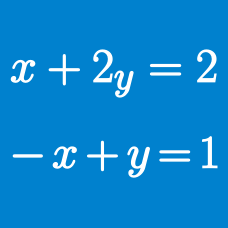Algebra

# System of Equations - Elimination

If $x, y$ are distinct values satisfying $x^2 = 21x + 12 y$ and $y^2 = 21 y + 12 x$, what is the value of $x^2 + y^2$?

What positive integer value of $M$ satisfies the following system of equations:

$\begin{cases} \sqrt{M} + \sqrt{N} = 25 \\ M - N = 175 ? \\ \end{cases}$

Let $a$ and $b$ be the values of real numbers $x$ and $y$, respectively, that satisfy $x^2-xy+y^2=3, x^2+y^2=5.$ What is the value of $\lvert{a+b}\rvert?$

Suppose $x$ and $y$ satisfy both of the nonlinear equations $\begin{cases} x^3 + y^3 & = 62 \\ x^2 - xy + y^2 & = 31. \end{cases}$ Find $xy$.

If $x=a \; (> 0)$ and $y=b$ are solutions of the simultaneous equations $9x^2-10xy+y^2=0,\, 5x^2-y^2=64,$ what is the value of $a+b$?

×